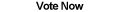Home Articles Components Code Snippets Links# C# Partition a List

## Partitioning a List

Partitioning a list in C#.NET means splitting a list of elements into smaller lists. How many lists are created or how big each partition is, depends on the context of each piece of source code.

There is no quick way to partition a list or an array, so we will explore two possible approaches.

## Number of Partitions

The first way is to write a C# function that will take a list (or array) and the number of partitions we want, and split the list accordingly. Which means in this case, we don't care how big each list is, we just want a certain number of partitions.

```public static List<T>[] Partition<T>(List<T> list, int totalPartitions)
{
if (list == null)
throw new ArgumentNullException("list");

if (totalPartitions < 1)
throw new ArgumentOutOfRangeException("totalPartitions");

List<T>[] partitions = new List<T>[totalPartitions];

int maxSize = (int)Math.Ceiling(list.Count / (double)totalPartitions);
int k = 0;

for (int i = 0; i < partitions.Length; i++)
{
partitions[i] = new List<T>();
for (int j = k; j < k + maxSize; j++)
{
if (j >= list.Count)
break;
}
k += maxSize;
}

return partitions;
}
```

The source code is nothing fancy. A single array holds each of the partitions (so we have an array of Lists). Each list is initialized and populated with a certain number of items from the original list. That certain number is the total number of elements divided by the total number of partitions we want. Note that the number is "rounded up" (with the Math.Ceiling function). This makes the distribution of the elements more densely concentrated in the first partitions.

For example, a list of 5 elements split into two partitions could result in 3 elements in the first partition and 2 elements in the second, or 2 elements in the first and 3 in the second. By "rounding up" we opt for the first case.

Also note the function works with C# List objects, it will not work with C# arrays. To make it work with arrays, just modify the code to use T[][] instead of List<T>[]. It is not too hard to do, so I will leave it as an exercise for you.

Keep in mind that it is possible to end up with empty partitions. If the original list has less elements that the total number of partitions requested, then there aren't going to be enough elements to go around. If you want to avoid empty sublists, then we need a different method...

## Size of Partitions

The second method is to instead give a C# function the original list and the maximum size of any each partition. The approach is very similar to the first method, but in this case we will never end up with empty partitions.

```public static List<T>[] Partition2<T>(List<T> list, int size)
{
if (list == null)
throw new ArgumentNullException("list");

if (totalPartitions < 1)
throw new ArgumentOutOfRangeException("totalPartitions");

int count = (int)Math.Ceiling(list.Count / (double)size);
List<T>[] partitions = new List<T>[count];

int k = 0;
for (int i = 0; i < partitions.Length; i++)
{
partitions[i] = new List<T>(size);
for (int j = k; j < k + size; j++)
{
if (j >= list.Count)
break;
}
k += size;
}

return partitions;
}
```

As you might imagine the C# source code is very similar. The main difference is at the beginning. Since we are now given the size of each partitions, we need to figure out how many partitions that will result in. Again we use the Math.Ceiling function because we do not want odd elements to be left out. The parameter is the max size, so the last partition can have less than the maximum size if the elements did not divide evenly.

## Conclusion

Splitting arrays in C# into smaller parts is useful in a number of different scenarios. It can allow users to view large collections of data in smaller pieces at a time. As concurrency becomes more prominent in .NET, breaking a large piece of data into smaller workable segments will become increasingly useful.

Chris went ahead and improved on the code quite a bit. He posted on his blog a way to partition lists in .NET 3.5, it is very well done and it is worth checking out.Back to C# Article List

Other C# Articles